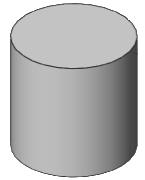# Cylinder Volume Calculator

The measurements that are inserted by the user will be used by the online Cylinder calculator to compute electronically the volume of the cylinder. The only things that are required to fill in are the radius of the base and the height of the cylinder for which the user needs to calculate the volume. The next action would be simply to click the "Calculate" window to carry out the process of calculation.

You will also have a running total which builds as you enter new dimensions into the volume calculator.

Enter the cylinder Base Radius and Height to calculate the volume of a cylinder.

## General information and basic definition of a cylinderA cylinder can be defined as a geometrical curvilinear shape that has three dimensions. The surface of a cylinder is formed by the points that are situated at a fixed distance from a given straight line which is the axis of the cylinder.

The following formula can be applied when calculating the Volume of the Cylinder:

• Base times Height (where Base is equal to π r2. r stands for Radius which is the distance from the central point to any point on the circumference. With the use of this equation the volume of a circle can be found).

Note: It is crucial that the user applies the same measurement units for all of the dimensions when working out the volume of any object.

Units used for measuring the volumes of solid objects are as follows:

• feet cubed
• meters cubed
• yards cubed

Units used for measuring liquids are:

• liters
• quarts
• pints
• gallons